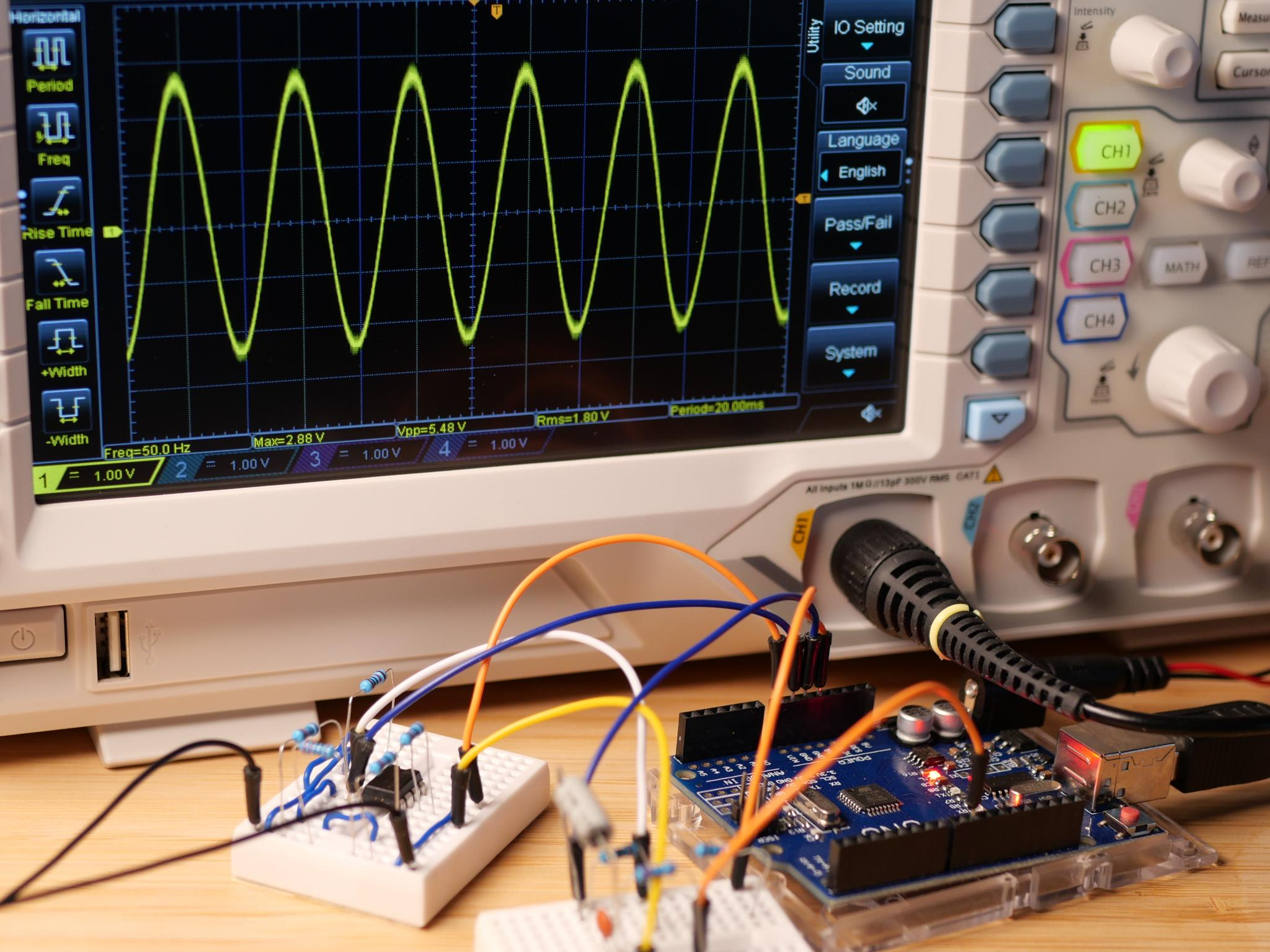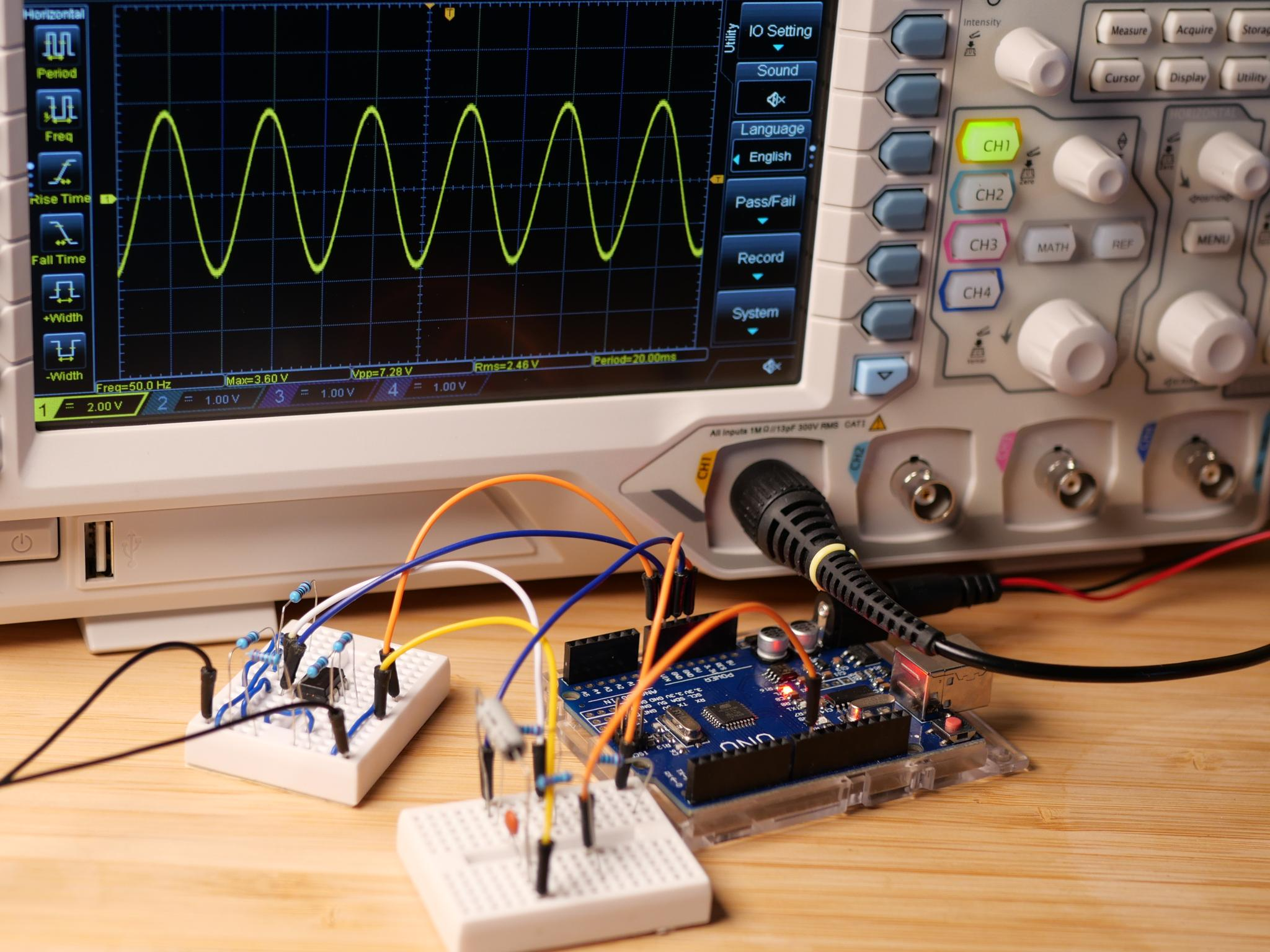# Low Voltage AC Source (Part 6)

In the last part of this project, we used an op amp to increase the current. This time we are going to increase the output voltage.

## Increasing the Output Voltage

In the last part of this project, we used an op amp to buffer the output of our signal generation circuit which allowed us to drive a 220 Ω load instead of the 10 kΩ we used before. As we were only interested in stabilizing the generated signal, we used the op amp in voltage buffer configuration. A voltage buffer has a voltage gain of one. It does not amplify the voltage. Today, we are going to see how an op amp can be used to also amplify the voltage of our signal.

## Op Amps as Non-Inverting Amplifiers

Let's start with the theory. The op amp circuit we are going to use to amplify our output signal is called a non-inverting amplifier. The circuit for such a non-inverting amplifier with a voltage gain of 2 is shown in the image below. But, how does it work?To understand this circuit, let's first revisit how the voltage buffer circuit worked. The op amp amplifies the voltage difference between the inverting (+) and the non-inverting input (-). It does so with a very high gain. However, by connecting the output to the non-inverting input we created a feedback loop. The op amp behavior changes. The amplified voltage difference is now the difference between the input voltage Vin and the output voltage Vout. If Vout is lower than Vin the op amp amplifies this positive voltage difference and the output voltage rises. For the opposite case the output voltage decreases. The stable state, that is reached after a very short amount of time, is the one, in which the input voltage is equal to the output voltage. In this state the voltage difference between both inputs is zero.

In the circuit of our non-inverting amplifier, the output is not directly connected to non-inverting input anymore. The two resistors R1 and R2 form a voltage divider. As both resistors have the same value it's easy to determine the division ratio: the voltage at the non-inverting input is half the output voltage. What does this mean for our amplifier circuit? Well, the principle is still the same: the stable state is the one in which there is no voltage difference between the op amps inputs. The difference is, that for reaching this state the output voltage needs to be twice as high as the input voltage (voltage gain of 2), as the voltage divider causes the voltage at the non-inverting input to be only half the output voltage. The amplification factor is determined by the voltage divider and can be calculated using the following formula:
$$A_v = 1 + {R1 \over R2}$$

One more thing: an op amp is not a step-up converter. An op amp cannot convert a low supply voltage in to a higher one. It can only amplify the signal by the given amplification factor, if its supply voltage is high enough. If it is not the amplifiers goes into saturation. The output voltage cannot be increased further and the output signal is cut-off at the top. As we know from the last part of this project, the maximum output voltage of the LM358 is around 1.5 V less than its supply voltage. This is why we used the additional 9V battery the last time. To make an output voltage of 5 V possible, we needed a higher supply voltage for the op amp. This time we are going to look at two different circuits one with 9 V supply voltage and one with 12 V. The higher the op amp's supply voltage, the higher the achievable voltage gain is.

## Building the Circuit

Let's start building the actual circuits. We are going to use the amplification circuit from above which gives us a voltage gain of 2. We need to build this circuit twice: one time for amplifying the generated signal and one time for our artificial ground reference. Let's get right into it and look at the full circuit and the required code modifications. We start with 9 V supply voltage and will then look at what is possible, if we further increase the supply voltage to 12 V. Once again, you can choose whether you want to use the MCP4725 module or the PWM DAC circuit to generate the sine wave.

### Using a 9 V Battery as Power Supply

Let's see what we need for the 9 V circuit. As we chose to use an amplification circuit with a voltage gain of 2, we need to be careful. The attempt to amplify the 5 V outputted by the signal generation circuit with a factor of 2 is of course deemed to fail. If we multiply 5 V by two we end up with 10 V. This is of course not possible with a supply voltage of 9 V. We could solve this issue by reducing the gain of the amplifier circuit. We, however, are going to choose an alternative method: limiting the output voltage range of our signal generation circuit in software.

How do we adapt the code for the 9 V supply voltage? The maximum output voltage of our op amp at 9 V is 7.5 V. However, the voltage of a 9 V battery is not always 9 V. It is less, if the battery is not fully charged. The truth is our circuit is also not exactly the most efficient and power saving one. Even if we connect a fresh 9 V battery the voltage won't stay at 9 V for very long. If we want the circuit to actually work, we should design it for a lower output voltage like 7 V or less. If we do so, we run into another problem: if keep using our 2.5 V that are amplified to 5 V as ground reference, the amplitude is limited to 2 V. We should use another voltage as ground reference. Luckily, we already solved this issue in the last part of this project. For the 5 V circuit we also moved the ground reference to another voltage. We exchanged one of the 220 Ω resistors with a 120 Ω resistor, which produced a voltage of roughly 1.75 V, which corresponds to 3.5 V after the amplification.

In the image below, you can see the complete circuit and the adjusted code for generating the sine wave values. The new code generates a sine wave between 0.5 V and 3.0 V. When amplified this corresponds to a sine wave between 1V and 6V. The amplitude is then 2.5 V.// Precalculate Sine Values between 0.5 V - 3.0V (25.5 - 153)
for(int i = 0; i < steps; i++) {
voltages[i] = sin(i*3.14*2/signalsteps) * 63.75 + 89.25;
}

In the image below you can see how the generated signal looks like on an oscilloscope. The amplitude shown on the oscilloscope is a bit higher, but this is only because of the noise generated by the power supply I used.### Using a 12 V Power Supply

While using a 9 V battery as power supply, we could see that the chosen voltage gain of 2 is a bit too high. We needed to reduce the amplitude of the generated sine wave in software. If we use a 12 V power supply, however, the situation improves.

In the image below you can see the circuit for 12 V. It uses 5 V as ground reference for the AC part of the circuit and the code is adjusted to generate a sine wave with an amplitude of 3.5 V. We are not using the full voltage range and an amplitude of 5 V after amplification to prevent the sine wave from getting cut-off at the bottom. The issue, that the op amp cannot sink a lot of current, if the voltage gets to close to ground, remains. The reason, why I chose to limit the voltage range a bit more and used 1.5 V instead of 1 V as the minimum output voltage is, that the current that flows to our 220 Ω load increases, if we increase the amplitude of our sine wave. In our 12 V example the current that flows at the sine waves minimum is $$I = {{5 V - 1.5 V} \over 220 Ω} \approx 16 mA$$. The op amp's maximum current of 10 mA at 1 V above ground would be insufficient.// Precalculate Sine Values 0.75 V - 4.25 V (38.25 - 216.75)
for(int i = 0; i < steps; i++) {
voltages[i] = sin(i*3.14*2/steps) * 89.25 + 127.5;
}

And here is how the generated signal looks on the oscilloscope:We were able to further increase the amplitude of the sine wave. We can achieve an even higher output voltage, by increasing the supply voltage and adjusting the resistor values in the amplification circuit to achieve a higher voltage gain. Beware, that the supply voltage for the Arduino Uno should not exceed 20 V. The recommended maximum supply voltage is 12 V, which is exactly what we built this second circuit for.

## The Result

With the external power supply and the new amplifier circuit we were able to create an AC signal with an amplitude of 3.5 V and an RMS voltage of roughly 2.5 V. By amplifying the voltage, we overcame the severe voltage range limitations of our previous circuit. Now, we can try to further increase the maximum output current using an external push-pull stage. This is going to be the topic for the next part of this project.